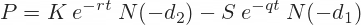# Fees (APR)

The protocol recalculates the put option premium daily, which becomes a borrower's accrued fee. We use the Black-Scholes Formula for this calculation.### APR % Breakdown

Below are the calculations for approximate APRs users would pay based on their loan-to-value ratio (risk).
Loan-to-Value (LTV)
APR (Yearly)
15%
~1%
30%
~16.5%
50%
~76%
Below are the inputs we currently use for the calculation. You can find all of our deployed contracts here, look for "PremiumPricer."
Input Variable
Value
S (Current NFT Average Floor)
Average NFT Floor Price
K (Strike or Borrow Amount)